# Quick ML

Simple code and explanations for the building block algorithms in ML.

A quick beginner's guide to coding the fundamental algorithms in ML has been on my todo-list for a while. Heavily inspired by Napkin ML

More coming soon!

This post hides some of the boilerplate code: if you want to extend these tutorials or play around with them, check them out here

### The Basic Imports¶

A machine learner never leaves home without NumPy

Warning: If you don't know NumPy, then this article may be difficult to comprehend. The Scipy Lectures are a good resource.

import numpy as np

### Introducing our Dataset¶

We'll use a simple 2 dimensional nonlinear dataset to run our algorithms, so that it's easier to visualize, yet complex enough that we see different types of behaviour from different algorithms.

# See Github for the full code - hidden for brevity Xs = ... Ys = ... test_Xs = ... test_Ys = ... def accuracy(model,X,Y): pass def plot(model,X,Y): pass

For each algorithm, we'll train it on a dataset with 1000 samples, and plot the decision curve learned by the algorithm. For example, here's the "optimal" classifier. The color of the point is the true class, and the color of the background is what the classifier predicts.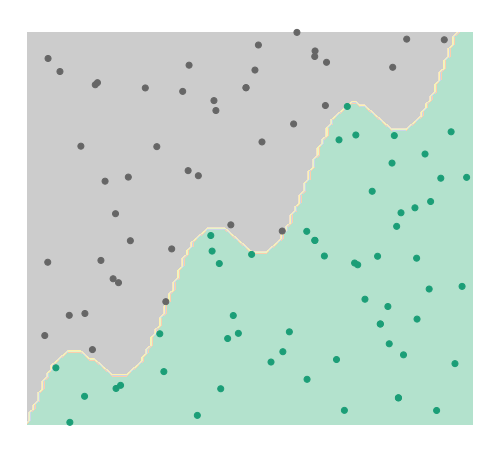## K-Nearest Neighbors¶

$k$-Nearest Neighbors is a non-parametric machine learning model that predicts classes for points x based on the k closest values to x seen in the training set.

from collections import Counter class kNN: def __init__(self,k=1): self.k = k def fit(self,xs,ys,k=1): """ Since kNN is nonparametric, we need to keep the training data around during test time. k-NN doesn't do any additional preprocessing """ self.xs = xs self.ys = ys def predict(self,x): # Sort training points by distance to the new point closest_points = np.argsort(np.linalg.norm(self.xs-x,axis=1))[:self.k] # Get labels of these close points closest_labels = self.ys[closest_points] # Return the most common label return Counter(closest_labels).most_common(1)
Accuracy is  0.978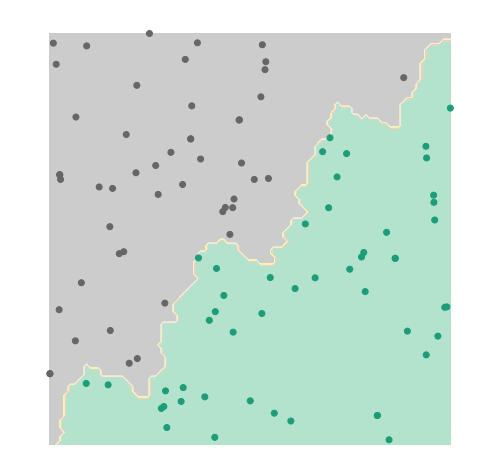## Linear Regression¶

Linear regression attempts to find the best linear model $f(x) =w^Tx$ to fit the data. The training procedure revolves around solving the following equation $$w^* = \arg\min_{w \in \mathbb{R}^d} \sum_{i=1}^n (w^TX_i - y_i)^2$$

We can write this in matrix form, where $X$ is a $n \times d$ matrix where each row corresponds to a training point. $$w^* = \arg\min_{w \in \mathbb{R}^d} \|Xw-y\|_2^2$$

Using matrix calculus, we have that $\nabla_w = X^T(Xw-y)$, so the optimal solution $w^*$ satisfies

$$(X^TX)w^* = X^Ty$$

class LinReg: def fit(self,xs,ys): """ Solving (X^TX)w^* = X^Ty """ self.w = np.linalg.solve(xs.T.dot(xs),xs.T.dot(ys)) def predict(self,x): return self.w.dot(x) > 0.5 # If predict >0.5, then say class 1, else class 0
Accuracy is  0.856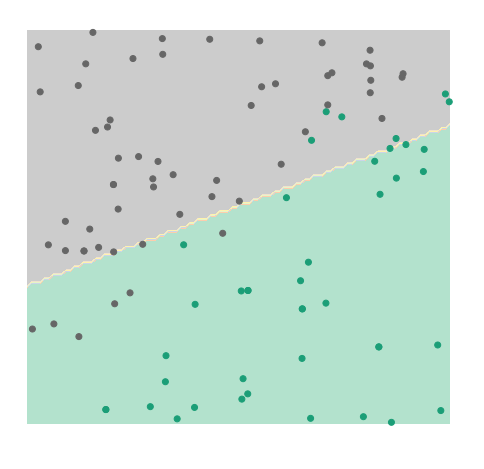## Logistic Regression¶

Logistic regression is similar to linear regression, but specializes to classification tasks by predicting probabilities $p(y_i=1~\mid~x_i)$.

If we naively apply linear regression to predicting probabilities, we'll be maximizing $p(y=1|x) = w^Tx$. However, since $w^Tx$ can be between $(-\infty, \infty)$, it matches poorly to the desired output $(0,1)$.

Estimating the odds $\cfrac{p(y=1~\mid~x)}{p(y=0~\mid~x)}$ as a linear function is better, but still attains values only in $[0,\infty)$. To truly exploit the full range of values that a linear function affords, we predict the log-odds as a linear function of $x$.

$$\text{Log-odds} = \log \frac{P(y=1|x)}{P(y=0|x)} = w^Tx$$

Solving this out for the probabilities, we see that the probability is given by the sigmoid function $$P(Y=1|x) = \cfrac{e^{w^Tx}}{1+e^{w^Tx}} = \frac{1}{1+e^{-w^Tx}}$$

We now have a model which predicts probabilities:

\begin{align*} P(Y=y ~~\vert~~X=x,w) &= \begin{cases} p & y=1 \\ 1-p & y=0 \end{cases}\\~\\ &=p^y(1-p)^{1-y} \end{align*}

Now, how do we find the so-called "best" probabilities? The standard method to do so is Maximum Likelihood Estimation (MLE), which maximizes the probability of the training data under our model. Intuitively, we would like to find the model which was most likely to generate the data in the first place.

In the following calculations, we'll use the abbreviation $p_i = \cfrac{e^{w^Tx_i}}{1+e^{w^Tx_i}}$ (our predicted probability for $y_i=1$ under $x_i$)

\begin{align*} w^* &= \arg \max_{w} \prod_{i=1}^n P(Y=y_i|X=x_i,w)\\ & \stackrel{(a)}{=} \arg \max_{w} \sum_{i=1}^n \log P(Y=y_i|X=x_i,w)\\ %&= \arg \max_{w} \prod_{i=1}^n \left(\frac{p_i}{1-p_i}\right)^{y_i}(1-p_i)\\ &= \arg \max_{w} \sum_{i=1}^n y_i \log \frac{p_i}{1-p_i} + \log 1-p_i \\ &= \arg \max_{w} \sum_{i=1}^n y_i w^Tx - \log 1+e^{w^Tx} \\ \end{align*}

Unlike linear regression above, this has no closed-form solution. To solve, instead we have two methods: gradient descent and convex optimization

The gradient of the objective is given by

$$\nabla_w = \sum_{i=1}^n y_i x - p_i x = \sum_{i=1}^n (y_i-p_i)x$$

class LogRegGD: def __init__(self,a=.001,nIter=1000): self.a = a # Learning Rate self.nIter = nIter # Number of gradient update def probabilities(self,xs): return 1/(1+np.exp(-1*xs.dot(self.w))) def fit(self,xs,ys): """ Take gradient steps """ xs = np.hstack([xs,np.ones((len(xs),1))]) # Add bias term self.w = np.random.rand(xs.shape) for i in range(self.nIter): ps = self.probabilities(xs) self.w += self.a*(ys-ps).dot(xs) # Take Gradient Descent term def predict(self,x): x = np.concatenate((x,)) # Add bias term return self.w.dot(x) > 0 # If w^Tx > 0, then p > .5
Accuracy is  0.941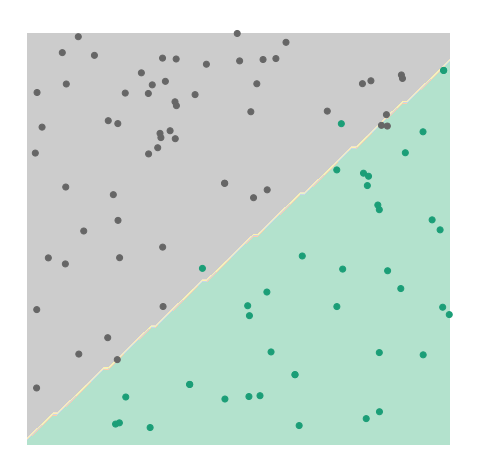### Convex Formulation¶

The objective function is convex; we can use a convex optimization library to solve. We'll use cvxpy (install guide here). This involves extra libraries, but has a significant advantage over the gradient descent objective in that it returns the exact solution, without worrying about hyperparameters and divergence concerns.

Recall from above that the objective is given by

$$\arg \max_{w} \sum_{i=1}^n y_i w^Tx - \log 1+e^{w^Tx}$$

We can write this in matrix notation as

$$y^TXw - \mathbf{1}^T\text{Logistic}(Xw)$$

Where $\mathbf{1}$ is the ones-vector in $\mathbb{R}^n$, and $\text{Logistic}(\cdot)$ applies the logistic function $f(x) = \log 1 + e^x$ to each entry

import cvxpy as cvx class LogRegCVX: def fit(self,xs,ys): # Adding a bias term xs = np.hstack([xs,np.ones((len(xs),1))]) # Formulating the objective w = cvx.Variable(xs.shape) objective = -1*(ys.dot(xs)*w-cvx.sum_entries(cvx.logistic(xs*w))) # Solving and storing the optimal value cvx.Problem(cvx.Minimize(objective)).solve() self.w = np.array(w.value).flatten() def predict(self,x): x = np.concatenate((x,)) # Add bias term return self.w.dot(x) > 0 # If w^Tx > 0, then p > .5
Accuracy is  0.935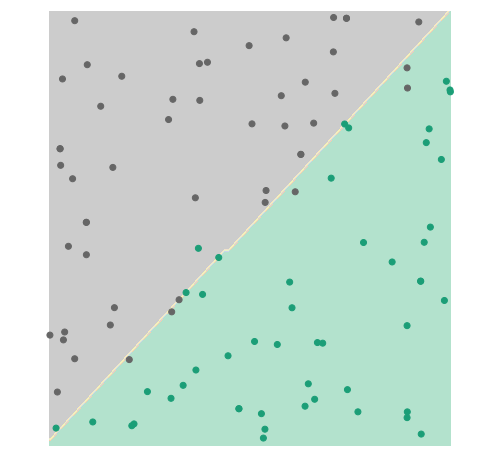Feel free to share!